# MONTH Function

Returns the month portion of a date as a number

## What is the MONTH Function?

The MONTH function is categorized under Excel DATE/TIME functions. The function will return the month portion of a date as a number. The number will be between 1 to 12. The MONTH function is available in all versions from MS Excel 2000.

In financial analysis, we can use MONTH with the DATE function to get desired results.

### Formula

=MONTH(serial_number)

The MONTH function uses only one argument:

Serial_number (required argument) – This is the date that you want to return the month of. The date can either be:

1. A serial number
2. Reference to cells containing dates
3. Date values returned from Excel formulas

### How to use the MONTH Function in Excel?

As a worksheet function, MONTH can be entered as part of a formula in a cell of a worksheet. To understand the uses of the function, let’s consider an example:

#### Example 1

Let’s see how this function works using the data below:

• 12/09/2017  9:40:00 AM
• 05/29/17
• 2015 June 7

The formula used is: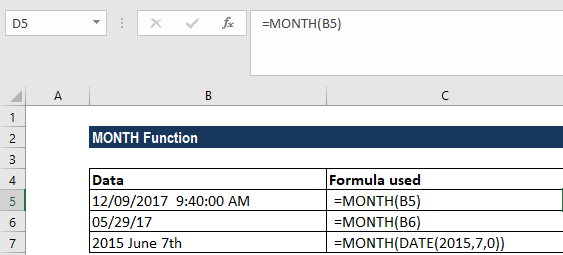We get the results below: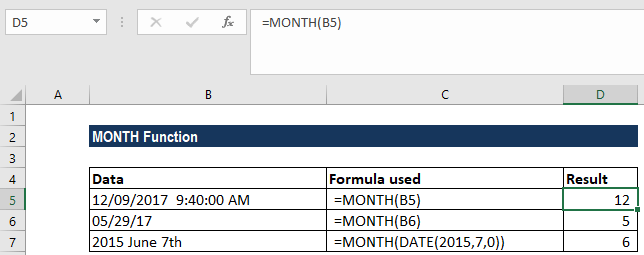In row A4, as the date is not in proper format, we used the DATE function along with the MONTH function.

#### Example 2

If we need to test two dates to check if they both are in the same month and year, we can do so with a simple formula that uses the MONTH and YEAR functions. Using the data below: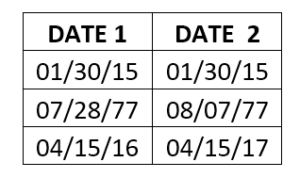The formula to be used is =MONTH(cell reference)&YEAR(cell reference), as shown below: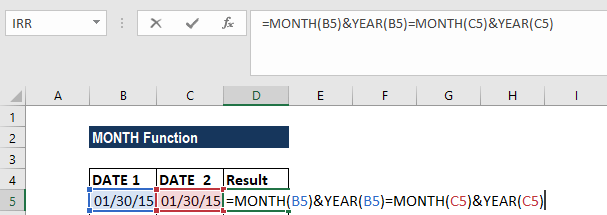In this case, Excel extracts the month from the date in cell A2 as numbers, then concatenates them together to form a text string. The same thing is done with the date in cell B2, the month and year are extracted and joined together.

Finally, the formula then tests if B2 is equal to C2 using the equal sign. Since both dates are in January 2015, the formula is solved as follows and returns TRUE for the first row. The results are shown below: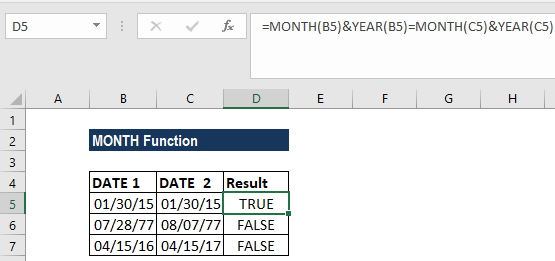Similarly, if we need to test a date to see if it is in the same month and year as the current date (today), you can use this formula:

=MONTH(date)&YEAR(date)=MONTH(TODAY())&YEAR(TODAY())

#### Example 3

We can even use this function to convert month names to numbers. For this, we need to use two functions DATEVALUE & MONTH.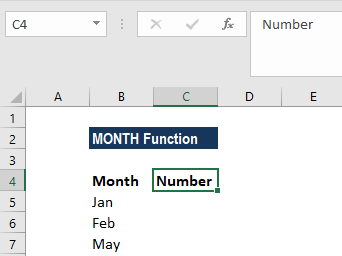The formula to use is =MONTH(DATEVALUE(A2 & “1”)).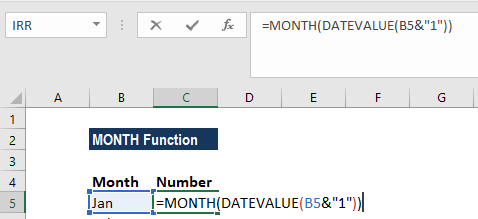We get the results below: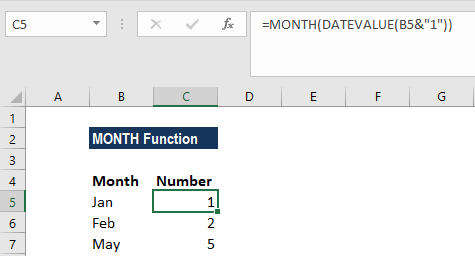### A few notes about the MONTH Function

1. Values returned by the YEAR, MONTH and DAY functions will be Gregorian values regardless of the display format for the supplied date value.
2. We can get results that look like a date – “01/01/18” – instead of an integer. This problem is most likely to be because the new cell or column is formatted as a ‘date.’ In this case, it is only the formatting of the cell that is wrong, NOT the value returned by the function. The easiest way to correct this is to select the General Formatting option from the drop-down format menu of the Excel ribbon. It is usually located in the ‘Number’ group, on the Home tab of the ribbon.
3. For the MONTH formula to work correctly, a date should be entered by using the DATE(year, month, day) function.

Thanks for reading CFI’s guide to important Excel functions! By taking the time to learn and master these functions, you’ll significantly speed up your financial analysis. To learn more, check out these additional CFI resources:

• Excel Functions for Finance